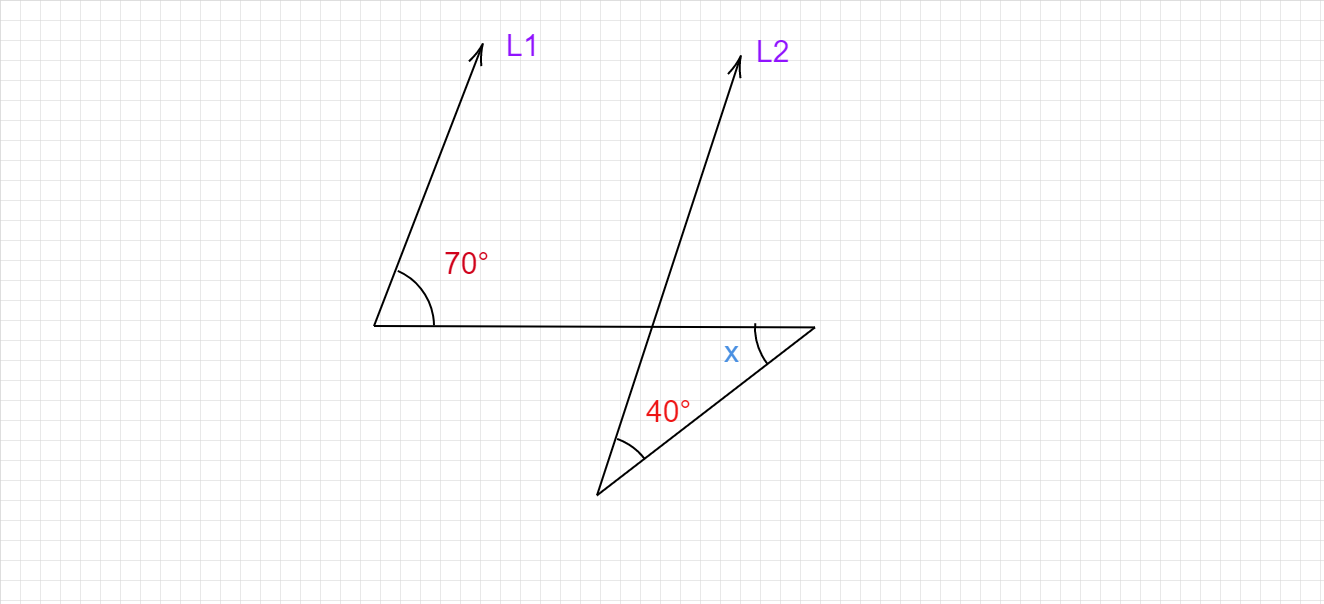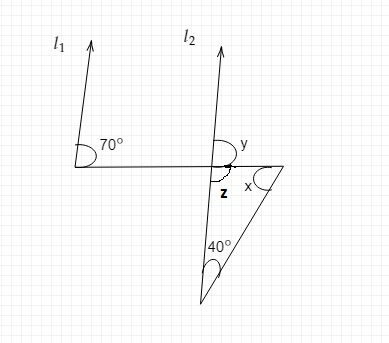"
">

# In the given figure L1 Parallel L2 , Find the value of x."

Given :

In the given figure the lines L1 and L2 are parallel to each other.

To do :

We have to find the value of x.

Solution :

We know that,

If two parallel lines are intersected by a transversal then the corresponding angles are equal.

Sum of the angles in a triangle is 180°.Let the angle corresponding to 70° be y as shown in the figure,

This implies,

$y = 70°$   (corresponding angles are equal)

$y+z = 180°$   (Linear pair)

$70°+z = 180°$

$z = 180°-70°$

$z = 110°$

$x+z+40° = 180°$

$x+110°+40° = 180°$

$x = 180°-150°$

$x = 30°$

The value of x is 30°.

Updated on: 10-Oct-2022

888 Views